# Solved 2022 Semester 2 Question Paper ICSE Class 10 Physics

## Section A (10 Marks)

#### Question 1(i)

Free vibrations are :

1. the vibrations under the influence of a periodic force.
2. the vibrations with larger amplitude.
3. the vibrations when the frequency continuously decreases.
4. the vibrations with a constant frequency and constant amplitude.

the vibrations with a constant frequency and constant amplitude

Reason — The periodic vibrations of constant amplitude and frequency of a body in the absence of any external force on it, are called free vibrations.

#### Question 1(ii)

The diagram below shows four sound waves. Which sound has the highest pitch?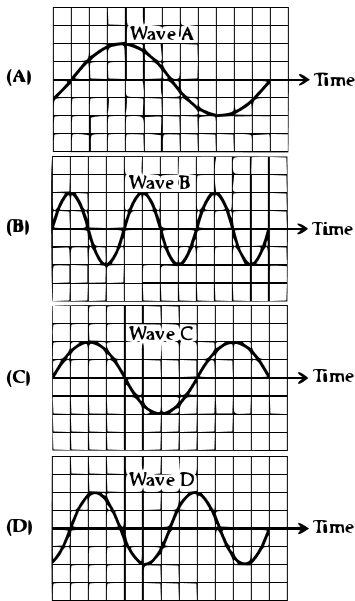Wave B

Reason — Pitch depends on frequency. Wave B has the highest frequency. So, it has the highest pitch.

#### Question 1(iii)

The graph plotted for potential difference (V) against current (I) for ohmic resistors is :

1. A curve passing through the origin.
2. A straight line not passing through origin.
3. A straight line passing through the origin.
4. A circle centred at the origin.

A straight line passing through the origin.

Reason — The relation between potential difference (V) and current (I) is I ∝ V. So, it is an equation of a straight line passing through the origin.

#### Question 1(iv)

A main switch in the main distribution board is present in :

1. a live wire
2. a neutral wire
3. a live as well as neutral wire
4. an earth wire

a live as well as neutral wire

Reason — The main switch is connected in both the live wire and neutral wires.

#### Question 1(v)

A conductor AB is kept along north south direction of the earth above a magnetic needle as shown below. When the key K is closed then: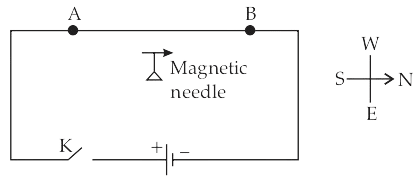1. the needle will not show any deflection.
2. the needle will deflect towards east.
3. the needle will turn in the opposite direction i.e. towards south.
4. the needle will deflect towards west.

the needle will deflect towards east.

Reason — As the key is closed, a current will flow through the wire and a magnetic field will be produced. In interaction with the earth’s magnetic field the resultant magnetic field will defect the needle towards east.

#### Question 1(vi)

A coil wound around a piece of soft iron can become an electromagnet only when:

1. the circuit is open.
2. a magnetic compass is present in the vicinity.
3. a galvanometer is connected to the circuit.
4. a current flows in the circuit.

a current flows in the circuit.

Reason — When the current flows through the soft iron piece in the form of a closed ring, it becomes magnetised.

#### Question 1(vii)

If water absorbs 4000 joule heat to increase the temperature of 1 kg water through 1°C then the specific heat capacity of water is :

1. 4 Jkg-1°C-1
2. 400 Jg-1°C-1
3. 4 Jg-1°C-1
4. 4.2 Jg-1°C-1

4 Jg-1°C-1

Reason — Specific heat capacity is the amount of heat energy required to raise the temperature of a substance per unit of mass. 4000 J heat is required to raise the temperature of 1 kg of water through 1°C i.e., 4000 J heat is required to raise the temperature of 1000 g of water through 1°C i.e., 4 J heat is required to raise the temperature of 1 g of water through 1°C. So, specific heat capacity of water is 4J g-1°C-1.

#### Question 1(viii)

Water is used in car radiators because :

1. it is a good conductor of heat.
2. it conducts heat faster as compared to the other substances and cools the engine quickly.
3. its specific heat capacity is very low.
4. its specific heat capacity is very high so it can cool the engine without a greater increase in its own temperature.

its specific heat capacity is very high so it can cool the engine without a greater increase in its own temperature.

Reason — Water in car radiators can absorb more heat without much rise in temperature due to it's high specific heat capacity. Hence, it acts as an effective coolant.

#### Question 1(ix)

The heaviest nuclear radiation is :

Reason — Alpha (α) radiation consists of alpha particles, which are composed of two protons and two neutrons. These particles are relatively large and heavy compared to other types of radiation, such as beta (β) and gamma (γ) radiation.

#### Question 1(x)

To study the age of excavated material of archaeological significance we study the rate of decay of an isotope of :

1. Uranium
2. Cobalt
3. Carbon
4. Chlorine

Carbon

Reason — The method of determining the age of archaeological material is known as Radiocarbon dating. It relies on the decay of the radioactive isotope carbon-14 to determine the age of organic materials.

## Section B (30 Marks)

#### Question 2(i)

The diagram below shows a magnetic compass kept closer to a coil AB wound around a hollow cylindrical cardboard :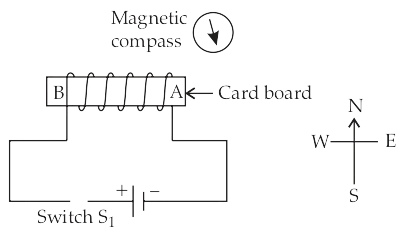(a) After studying the circuit and the magnetic compass carefully, state whether the switch S1 is open or closed.

(b) How did you arrive at the conclusion in (a)?

(c) What is the purpose of placing the magnetic compass in the above setup?

(a) The switch S1 is closed.

(b) When the key is pressed, a current passes in the wire in the direction from A to B (i.e. from south to north) and the north pole (N) of the needle deflects towards the west. There is a deflection in the magnetic compass. So, it is concluded that the switch S1 is closed and current is flowing through the coil.

(c) The purpose of placing the magnetic compass is to determine the polarity developed at the end A of the solenoid.

#### Question 2(ii)

(a) Give an important reason for copper to be used as a material for a calorimeter.

(b) Calculate the thermal capacity of 40 g of water.

[Specific heat capacity of water = 4200 JKg-1°C-1]

(a) The reasons for using copper is that it has low specific heat capacity so the heat capacity of calorimeter is low and the amount of heat energy taken by the calorimeter from the contents to acquire it's temperature, is very small.

(b) Thermal capacity = specific heat capacity x amount of substance

∴ Thermal capacity of 40 g water = 4200 x $\dfrac{40}{1000}$ = 168 J° C-1

#### Question 2(iii)

In the below circuit diagram, calculate:

(a) the external resistance of the circuit.

(b) the current I2.

(c) the current I.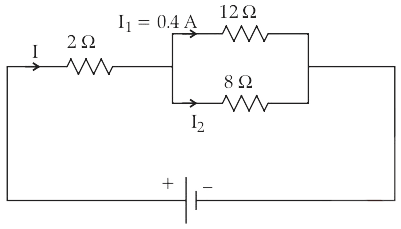(a) 12Ω and 8Ω are in parallel.

$\dfrac{1}{\text{R}}$ = $\dfrac{1}{12}$ + $\dfrac{1}{8}$ = $\dfrac{2+3}{24}$ = $\dfrac{5}{24}$

So the equivalent resistance = $\dfrac{24}{5}$ = 4.8Ω

2Ω is in series with this combination.

Hence the total resistance = 2 + 4.8 = 6.8Ω

(b) Potential difference across the 12Ω resistor = potential difference across 8Ω resistor

Or, 12 × 0.4 = 8 × I2

∴ I2 = $\dfrac{12 × 0.4 }{8}$ = = 0.6 A

(c) Current I = I1 + I2 = 0.4 + 0.6 = 1 A

#### Question 3(i)

Three wires with proper colour coding are connected to the three terminals of a three-pin socket. Match the colour of the wire with the proper terminals A, B and C of the socket.(a) Brown

(b) Green

(c) Light blue

(a) Brown → C

(b) Green → A

(c) Light blue → B

#### Question 3(ii)

(a) Why does it become colder after a hailstorm than during or before the hailstorm?

(b) 'If two bodies have the same specific heat capacities, then they will always absorb the same amount of heat if their temperature increases by the same amount.' State whether the given statement is true or false.

(a) It is generally cold after a hail storm than during and before it because after the hail storm, ice absorbs the heat energy required for it's melting from the surroundings, so the temperature of the surrounding falls further down and we feel more cold.

(b) The given statement is false. Heat energy absorbed by a body is directly proportional to the mass of the body i.e., Q ∝ m also. So, if the masses of the two bodies are different then they will absorb different amounts of heat.

#### Question 3(iii)

A metal piece of mass 420 g present at 80°C is dropped in 80 g of water present at 20°C in a calorimeter of mass 84 g. If the final temperature of the mixture is 30°C, then calculate the specific heat capacity of the metal piece.

[Specific heat capacity of water = 4.2 Jg-1 °C-1, Specific heat capacity of the calorimeter = 200 Jkg-1 °C-1]

Given,

Mass of metal = 420 g at temperature = 80°C

Mass of water = 80 g at temperature = 20°C

Sp. Heat of water = 4.2 J g-1 °C-1

Mass of calorimeter = 84 g

Sp. heat of calorimeter = 200 J kg-1 °C-1 = 0.2 J g-1 °C-1

Final temperature of mixture = 30°C

Sp. Heat of metal [S] = ?

Heat energy lost by metal
= mass of metal x specific heat capacity x fall in temperature
= 420 x S x (80-30)
= 420 x S x 50
= 21000 S J

Heat energy gained by water
= mass of water x specific heat capacity x rise in temperature
= 80 x 4.2 x (30-20)
= 80 x 4.2 x 10
= 3360 J

Heat energy gained by calorimeter
= mass of calorimeter x specific heat capacity x rise in temperature
= 84 x 0.2 x (30-20)
= 84 x 0.2 x 10
= 168 J

As, heat energy lost = heat energy gained

∴ 21000S = 3360 + 168

∴ S = $\dfrac{3528}{21000}$ = 0.168 J g-1 °C-1

Hence, Sp. Heat of metal = 0.168 J g-1 °C-1

#### Question 4(i)

Rohit playing a flute and Anita playing a piano emit sounds of same pitch and loudness.

(a) Name one characteristic that is different for waves from the two different instruments.

(b) If now the loudness of the sound from flute becomes four times that of the sound from piano, then write the value of the ratio AF : AP (AF – amplitude of sound wave from flute, AP – amplitude of sound wave from piano)

(c) Define 'Pitch' of a sound.

(a) The characteristics is quality.

(b) Loudness (L) is proportional to the square of amplitude (A)

So,

$\dfrac{\text{L}_F}{\text{L}_P}$ = $\dfrac{\text{A}_F^2}{\text{A}_P^2}$

Or, $\dfrac{4}{1}$ = $\dfrac{\text{A}_F^2}{\text{A}_P^2}$

$\dfrac{\text{A}_F}{\text{A}_P}$ = 2:1

(c) Pitch is that characteristic of sound by which an acute (or shrill) note can be distinguished from a grave (or flat) note of same loudness.

#### Question 4(ii)

(a) Name two factors on which the force experienced by a conductor carrying current, placed in a magnetic field, depends. Also, state how these factors affect the force.

(b) With the help of which rule you can determine the direction of force acting on a current carrying conductor placed in a magnetic field?

(a) Experimentally, it is found that the magnitude of force acting on a current carrying conductor placed in a magnetic field in the direction perpendicular to it's length, depends on the following factors —

1. The force F is directly proportional to the strength of magnetic field B, i.e., F α B
2. The force F is directly proportional to the current I flowing in the wire, i.e., F α I

(b) Fleming's left hand rule.

#### Question 4(iii)

(a) What is nuclear energy?

(b) After emission of a nuclear radiation, the atomic number of the daughter nucleus increases by 1. Identify the nuclear radiation.

(c) Write a nuclear reaction indicating the nuclear change mentioned in (b).

(d) What is the special name given to the parent and daughter nucleus when this radiation is emitted?

(a) Nuclear energy arises from radioactive processes like decay, fission, or fusion. During these changes, the combined mass of product nuclei becomes less than that of the reactant nuclei, resulting in a mass loss. This mass loss leads to liberation of energy which is known as nuclear energy.

(c) The nuclear reaction for β-radiation is given below:

$\begin{matrix} _{Z}^{A}\text{P} & \longrightarrow & _{Z+1}^{\space \space \space \space \space A}\text{Q} & + & \space _{-1}^{\space \space \space 0}\text{e} \\ \footnotesize{\text{Parent nucleus}} & & \footnotesize{\text{Daughter nucleus}} & & \footnotesize{\text{(Beta-particle)}} \end{matrix}$

(d) Isobars.

#### Question 5(i)

An appliance rated 440 W, 220V is connected across 220V supply.

(a) Calculate the maximum current that the appliance can draw.

(b) Calculate the resistance of the appliance.

(a) Maximum current drawn = I = $\dfrac{\text{W}}{\text{V}}$ = $\dfrac{440}{220}$ = 2A

(b) Resistance of the appliance = R = $\dfrac{\text{W}}{\text{I}^2}$ = $\dfrac{440}{4}$ = 110Ω

#### Question 5(ii)

The diagram below shows a vibrating tuning fork E mounted on a sound box X. When the vibrating tuning forks A, B, C and D are placed on the sound box Y one by one, it is observed that a louder sound is produced when the tuning fork B is place on Y.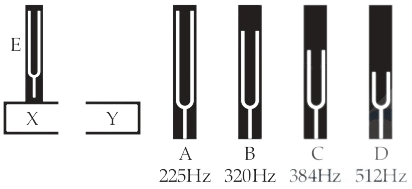(a) What is the frequency of tuning fork E?

(b) Why does B produce a louder sound?

(a) Frequency of E is 320 Hz.

(b) B produces large sound since the frequency of B matches with the frequency of E and resonance occurs.

#### Question 5(iii)

(a) From the graph of heating curve given below state the melting point and boiling point of the substance.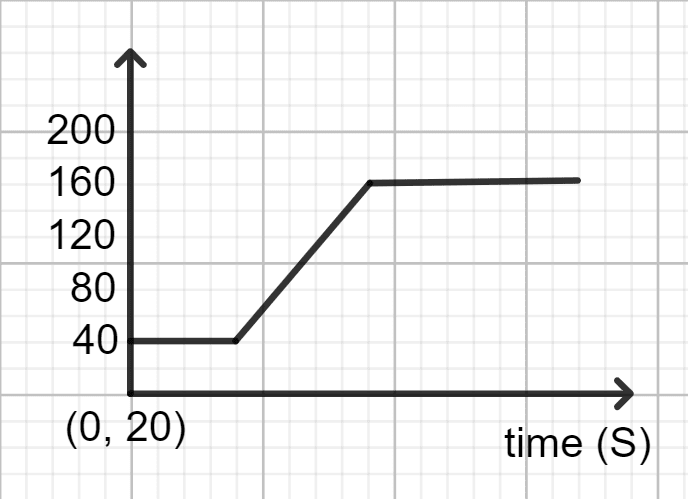(b) Complete and rewrite the following nuclear reaction by filling in the blanks.

235U ⟶ + 90Th + 42He

(a) Melting point is 40°C and boiling point is 160°C.

(b) 23592U ⟶ 23190Th + 42He

#### Question 6(i)

Study the below figure and answer the following :

(a) What type of vibration does the figure represent?

(b) State one reason for which the amplitude of the vibration decreases with time.

(c) Write an example of natural vibrations.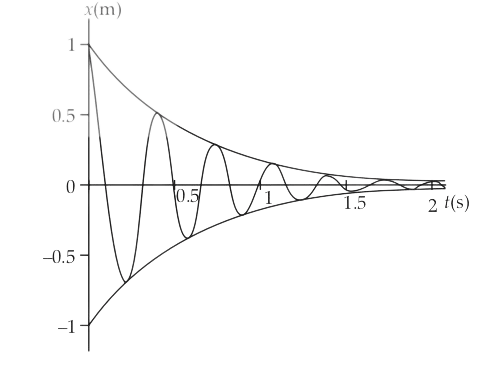(a) It is damped vibration.

(b) The surrounding medium offers resistance (or friction) to the motion, so the energy of vibrating body continuously decreases due to which the amplitude of vibration gradually decreases.

(c) A body clamped at one point when disturbed slightly from its rest position, starts vibrating. The vibrations so produced are called the natural or free vibrations of the body.

#### Question 6(ii)

A certain beam of α particles, β particles and γ radiations travel through a region of electric field produced between two oppositely charged parallel plates A(+) and B(–).

(a) Which of the above three has the maximum speed?

(b) Which one deviates the most from its original path?

(c) Which one does not deviate at all when passing through a region of electric or magnetic field?

(b) β particles.

#### Question 6(iii)

If a wire of resistance 2Ω gets stretched to thrice its original length :

(a) Calculate the new resistance of the wire.

(b) What happens to the specific resistance of the wire?

(a) Given, R = 2Ω

⇒ ρ$\dfrac{\text{l}}{\text{A}}$ = 2Ω

Let new resistance be R'.

New length = $3\text{l}$

New Area = $\dfrac{\text{A}}{3}$

∴ R' = ρ$\dfrac{\text{3l}}{\dfrac{\text{A}}{3}}$

= ρ$\dfrac{\text{9l}}{\text{A}}$

= 9R = 9 × 2 = 18Ω

Hence, new resistance = 18Ω

(b) Specific resistance of the wire remains unchanged since it does not depend on the dimensions of the wire. It is the characteristic property of the substance.